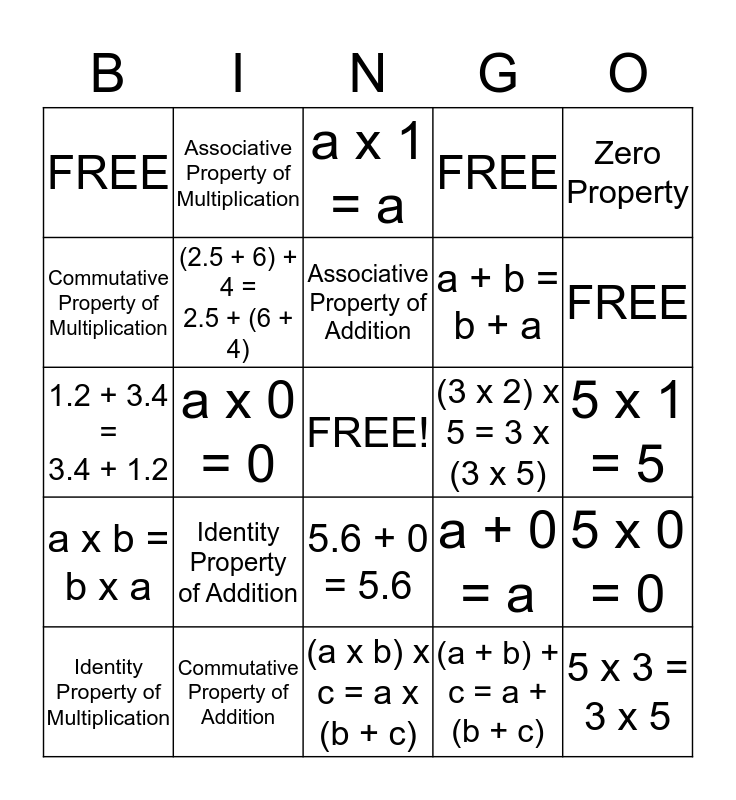# Property BINGOThis bingo card has a free space and 24 words: Identity Property of Addition, Commutative Property of Addition, Associative Property of Addition, Identity Property of Multiplication, Zero Property, FREE, Commutative Property of Multiplication, Associative Property of Multiplication, (3 x 2) x 5 = 3 x (3 x 5), 5.6 + 0 = 5.6, a + b = b + a, 1.2 + 3.4 = 3.4 + 1.2, a + 0 = a, 5 x 0 = 0, (2.5 + 6) + 4 = 2.5 + (6 + 4), (a + b) + c = a + (b + c), 5 x 1 = 5, a x 1 = a, FREE, a x 0 = 0, 5 x 3 = 3 x 5, a x b = b x a, FREE and (a x b) x c = a x (b + c).

⚠ This card has duplicate items: FREE (3)

## Play Online